1) Distance and the Physical Size of a Celestial Object

When we look up in the sky, we can only measure the angular size of the objects we see. For example, Mars may appear to have a radius of 10 arcseconds. The Andromeda galaxy has a radius of about 1.5 degrees. We need to know the distance to the object to convert the angular size into a physical size (using the trigonometric function tangent).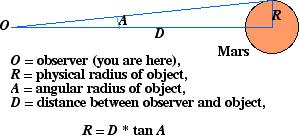In this example, if A = 10 arcsec and D = 70,000,000 km, so we discover that R = 3,400 km. We now know how large Mars is, and we can compare it to the Earth and other planets in the solar system.

2) Distance and the Luminosity of an Object

How bright an object appears to us depends on its distance. For example, a 100 watt lightbulb looks very bright when observed from a distance of a few feet, but is barely visible from a distance of one mile. Similarly, a star with a luminosity like that of the Sun (1 Lsun) appears very bright from the distance of 1 astronomical unit, but is almost invisible to the naked eye when observed at a distance of 10 parsecs. The distance-luminosity formula relates the apparent brightness of an object (b) with its luminosity (L) and distance (D):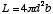In practice, astronomers traditionally use the magnitude system for measuring star brightnesses. We refer to the apparent magnitude (m) of an object instead of its apparent brightness, and the absolute magnitude (M) instead of its luminosity. The difference is called the distance modulus, and is defined as: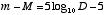So, we measure the apparent magnitude with our telescope and camera system. If we know the distance, we can determine the object's absolute magnitude (or luminosity). We thereby learn how much energy an object is emitting. This was critical in understanding how stars create energy through nuclear fusion in their cores.

3) Distance and Stellar Mass

Some stars exist in pairs called binaries. The stars orbit around each other, bound together by their mutual attraction due to gravity. Kepler's Third Law (and a few clever tricks) enables us to measure the mass of each star (M1 and M2; don't confuse them with magnitudes!) from the average distance between the two stars (a) and the period of time it takes to complete one orbit (P):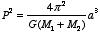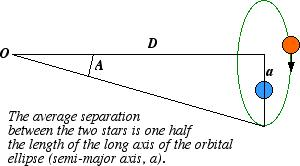where G is the gravitational constant. To measure a, astronomers follow the method of Part 1 to convert an angular separation (A) between the two stars (usually a few arcsec) into a physical separation in kilometers.

Knowing the masses of stars enables us to understand more about their nature. For example, if we know the mass of a star, we can make a computer model that describes the interior structure of the star by using a number of physical laws relating gas pressure, bouyancy, etc. The model tells us the density, temperature, and pressure at any place in the star, as well as how much energy is being produced by nuclear fusion at different places. It enables us to figure out how stars work. Most importantly, it tells us how stars change (evolve) as they run out of fuel. Much of what we know about the interesting late stages in stars' lives (red giants, planetary nebulae, supernovae, white dwarfs, neutron stars) has been learned through computer modelling. Observations of stellar masses enable us to create realistic models.

Determining the distance to a star, planet, or galaxy is therefore fundamental to learning pretty much anything at all about the object: its physical size, the amount of light it produces (or reflects), and its mass. Indirectly, theories like stellar evolution are based on distance measurements, and stellar evolution theory enables us to measure things like the ages of star clusters. More complicated concepts and descriptions are generated from more basic ones. Our understanding of the cosmos is a complex web of these ideas, but one of the most central parts is the knowledge of celestial distances.

4) Interstellar Reddening and Distances

Interstellar dust absorbs starlight and makes stars appear fainter. This messes up distance-finding methods like standard candles, unless we have a means to determine and correct for the absorption (AV -- absorption in the V wavelength band). The distance modulus equation expands to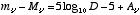where the V subscripts specify we are talking specifically about quantities measured in the wavelength range of the V filter. Solving for D, the equation looks like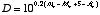.

How do we determine AV? A good way is from the amount of reddening -- since the interstellar dust causes them both, they are usually related: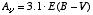where E(B-V) is called the color excess. It is simply the difference between the observed (B-V) color of the star minuts the star's intrinsic (or un-reddened) color, (B-V)0,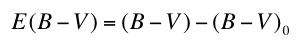.

The color is the apparent magnitude of the star observed through a B (blue) filter minus the magnitude observed through a V (visual, greenish) filter. For example, a star may have a visual magnitude of V = 12.31 mag and B = 12.67 mag, giving it a color index of (B-V) = 12.67 - 12.31 = 0.36 mag. In our filter system, there are also an ultraviolet filter (U), a red filter (R), and an infra red filter (I) -- UBVRI. One can create a color index using any pair of filters, for example (U-B), and (V-R). Our study focuses on the (V-I) color. Larger color index indicates a star with a redder, cooler surface: (V-I) = 0.2 mag is rather blue/hot, while (V-I) = 2.2 is quite red/cool.

For example: if a star is observed to have (B-V) = 0.84 mag and E(B-V) = 0.16 mag, we know that the star's intrinsic color must be (B-V)0= (B-V) - E(B-V) = 0.84 - 0.16 = 0.68 mag. We also know the star has been dimmed by AV = 3.1 x 0.16 = 0.496 = 0.50 mag. We can then compute its distance in parsecs (1 pc = 3.26 light years) using the D = 10^0.2(mV - MV + 5.0 - AV) equation if we have measured mV and somehow know MV.

5) Note: There are no truely un-reddened RRL. Even at the Galactic poles, there seems to be about 0.02 mag of reddening. We will focus on RRL at high latitude (40 degrees or more from the Galactic Plane), and then use the Schlegel et al. (1998) maps to determine the reddening E(V-I) of each star and make a small reddening correction to our observed (V-I) to arrive at a good estimate of each star's intrinsic color, (V-I)0.

6) Glossary

Apparent Brightness, Apparent Magnitude: How bright an object appears to us. The units of apparent brightness are those of flux: for example ergs/sec/cm2. Apparent magnitudes are measured on the magnitude scale: the star Vega has a magnitude of 0, the faintest stars you can see with the unaided eye are about magnitude 5, the RR Lyrae we observe with the BGSU telescope have magnitudes between 11 and 13, and the faintest objects observable by the biggest telescopes on earth are about 28. We often refer to the apparent magnitude observed through a specific filter; for example, V = 12.52 is the apparent magnitude measured through the visual (or "V") filter. Magnitude is a logarithmic scale. The magnitude difference between two stars is defined as: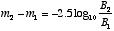where B indicates apparent brightness. So, if Star 2 is two times brighter than Star 1, it will have a magnitude 0.75 mag smaller than that of Star 1 (-2.5*log (2/1) = -0.753). A magnitude difference of 5 corresponds to a brightness ratio of 100, while a magnitude difference of 10 corresponds to a brightness ratio of 10,000!

Arcminute, Arcsecond: Units for measuring angular size. A circle is broken into 360 degrees. Each degree is broken into 60 arcminute, and each arcminute is broken into 60 arcseconds. Thus there are 60 arcsec/arcmin * 60 arcmin/deg = 3600 arcsec in a degree. For reference, the moon has an angular diameter of about 0.5 deg, or 30 arcmin, or 1800 arcsec.

Luminosity, Absolute Magnitude: The intrinsic brightness, or energy output of a celestial object. Light bulbs come marked with different wattages, meaning how much light energy they emit. The light energy output of stars is sometimes measured in solar luminosities (Lsun). Observers often refer to the absolute magnitude of an object: the apparent brightness it would have if it were observed from a distance of 10 parsecs. The Sun has an apparent magnitude of about 4.7, which means it would be barely visible to the naked eye if seen from a distance of 10 pc.

Andy Layden (layden@baade.bgsu.edu), January 2001 -- Back to Variable Star Project Page.